# Exercise 1.4 Number Systems NCERT Solutions Class 9

Go back to  'Number Systems'

## Chapter 1 Ex.1.4 Question 1

Visualize \begin{align} 3.765\end{align} on the number line, using successive magnification.

### Solution

Steps:

(i) $$3.7$$ lies between $$3$$ & $$4$$. So we divide the portion between $$3$$ & $$4$$. on the number line into $$10$$ equal parts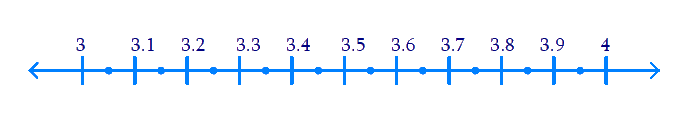$$3.76$$ lies between $$3.7$$ and $$3.8$$. So, we divide the portion between $$3.7$$ and $$3.8$$ on the number line into $$10$$ equal parts.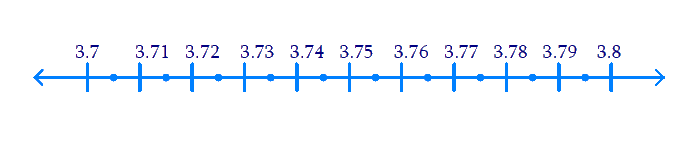$$3.765$$ lies between $$3.76$$ and $$3.77$$ and $$3.77$$ .Dividing the line before we got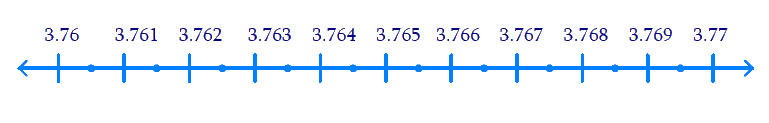## Chapter 1 Ex.1.4 Question 2

Visualize \begin{align}4 . \overline{26}\end{align} on the number line, up to $$4$$ decimal places.

### Solution

Steps:

\begin{align}4 . \overline{26}=4.2626\dots\end{align}

(i) $$4.2$$ lies between $$4$$ &$$5$$. Observe the number line given below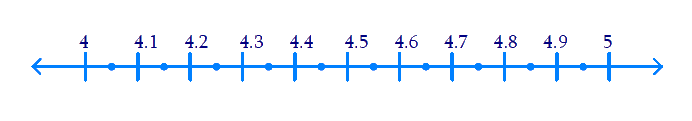(ii) $$4.26$$ lies between $$4.2$$ & $$4.3$$, shown below on the number line.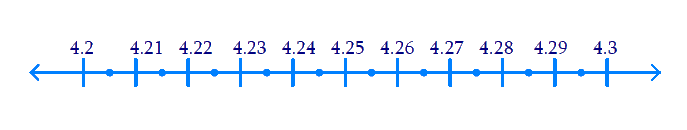(iii) $$4.262$$ lies between $$4.26$$ & $$4.27$$ as shown below.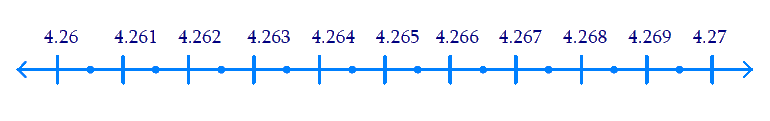(iv) $$4.2626$$ lies between $$4.262$$ & $$4.263$$ as shown below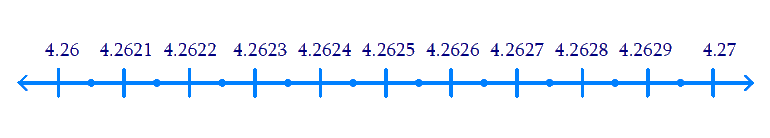Hence by the successive magnification method required number is obtained in the number line.

Related Sections
Related Sections
Instant doubt clearing with Cuemath Advanced Math Program